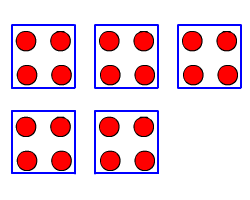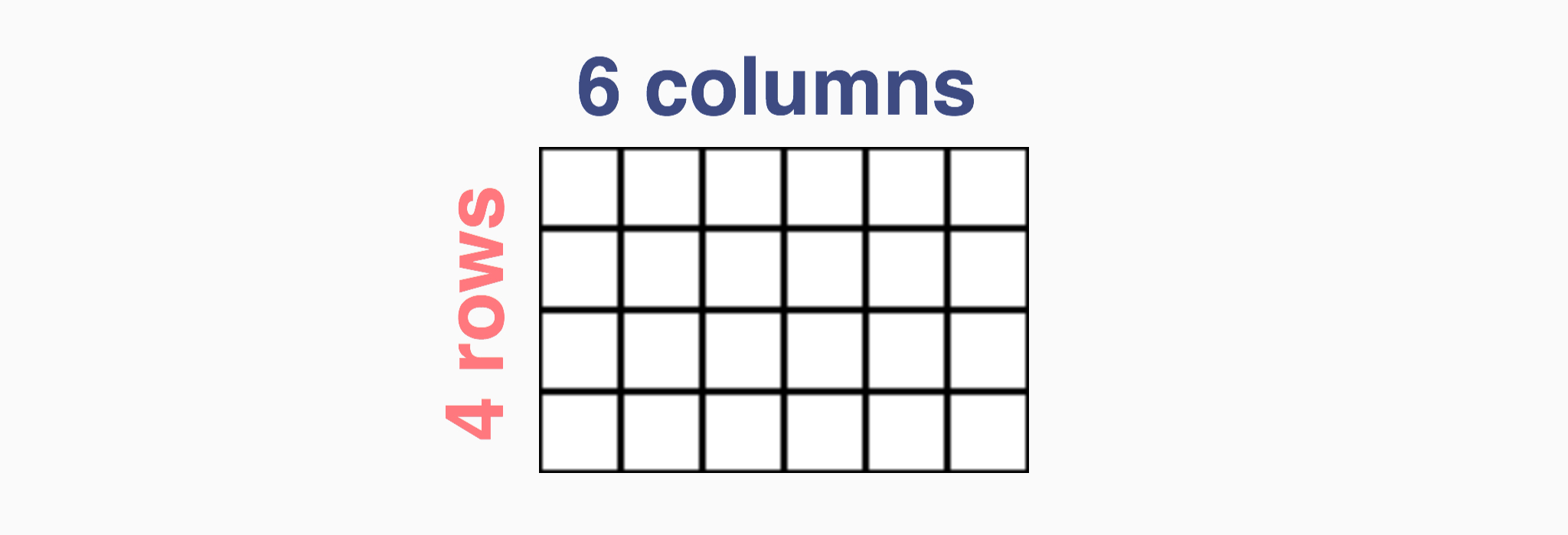Skip Counting
Multiplication Strategies
True or False
Multiplication Word Problems
Division Word Problems
100

Katie's class is counting around by 5. What number does the 10th student say?

50

100

Which multiplication equation can you use to find 3 + 3 + 3 + 3 + 3?

5 X 3 = 15

100

The multiples of 10 all end in 0. True or false?

True

100

George has 3 folders. He puts 2 papers in each folder. How many papers are there in all?

6 papers

100

Mitch has 10 dog toys. He gives 2 dog toys to each dog. How many dogs get dog toys?

5 dogs

200

Joe's class is counting around by 4. What number does the 9th student say?

36

200

Draw equal groups to represent 5 X 4.200

The multiples of 5 all end in 0 or 2. True or false?

False

200

Carol has 5 shelves. She puts 5 books on each shelf. How many books are there in all?

25

200

Lauren's mother baked 72 cookies for her friends. Lauren put the cookies in bags. She put 9 cookies in each bag. How many bags of cookies did she make?

8 bags

300

Kim's class is counting around by 3. What number does the 7th student say?

21

300

Which multiplication equation can you use to find 6 + 6 + 6? YOU MUST GIVE THE PRODUCT ALSO.

3 X 6 = 18

300

_____ X 8 = 80

The missing factor is 10. True or false?

True

300

A spider has 8 legs. How many legs are on 3 spiders?

24 legs

300

There were 16 dog legs in the backyard. Each dog has 4 legs. How many dogs were in the backyard?

4 dogs

400

Bob's class is counting around by 9. What number does the 7th student say?

63

400

Draw an array to represent 4 X 6.400

The multiples of 3 all odd numbers. True or false?

False

400

How many legs are on 12 humans?

24 legs

400
Tom has 24 stickers. He puts them on 4 pages to save them for his collection. Which operation can you use to find how many stickers are on each page?

24 divided by 4 = 6

500

Susan's class is counting around by 7. What number does the 11th student say?

77

500

If I start at 0 and skip count by 3, what number will I NOT count?

6, 13, 15, 9

13

500

6 X ____  = 42

The missing factor is 7. True or false?

True

500

Noah had 12 ice cream sundaes. He put 5 cherries on each ice cream sundae. How many cherries did he use in all?

60 cherries

500

Zane paid $33 dollars for 3 hats. Each hat cost the same amount. How much did each hat cost?$11.00

Click to zoom